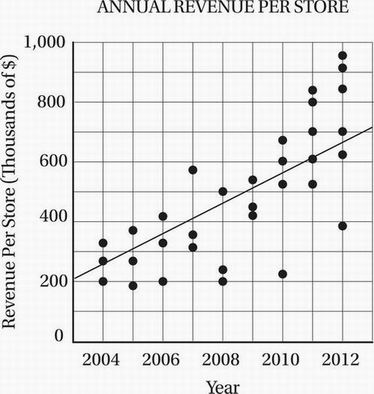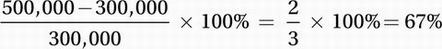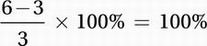# SAT Math Multiple Choice Question 670: Answer and Explanation

### Test Information

Question: 670

10.Which of the following statements is most directly justified by the data shown in the scatterplot above?

• A. The average revenue per store increased by over 100% from 2005 to 2009.
• B. The total number of retail stores increased by 50% from 2005 to 2012.
• C. The total revenue from all stores in 2012 was more than three times the total revenue from all stores in 2004.
• D. The total revenue from all stores in 2008 was over \$1 million.Explanation:

C

Data Analysis (scatterplot) MEDIUM-HARD

When faced with a question like this, we must analyze each statement individually.

(A) The average revenue per store increased by over 100% from 2005 to 2009. True or false? In 2005, according to the line of best fit, the average revenue per store was approximately \$300,000. In 2009, the average revenue per store was approximately \$500,000. This is a percent increase of(B) The total number of retail stores increased by 50% from 2005 to 2012. True or false? According to the scatterplot, in 2005 there were 3 stores corresponding to the three dots above 2005. In 2012 there were 6 stores corresponding to the 6 dots above 2012. This is a percent increase ofFALSE

(C) The total revenue for all stores in 2012 is more than three times the total revenue from all stores in 2004. True or false? In 2004, there were 3 stores with an average revenue per store of approximately \$250,000. Therefore the total revenue in 2004 was approximately 3 × \$250,000 = \$750,000. In 2012, there were 6 stores with an average revenue per store of approximately \$650,000. Therefore the total revenue in 2012 was approximately 6 × \$650,000 = \$3,900,000. Since \$3,900,000 is more than three time \$750,000, this statement is TRUE.